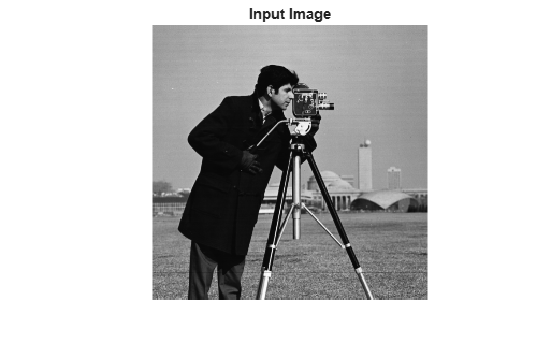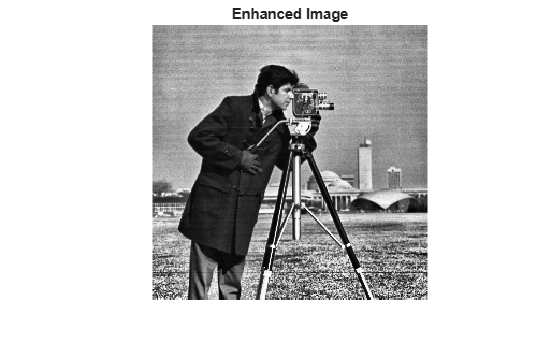# getBasePtr

Create MATLAB interface object for OpenCV base class

Since R2021b

## Syntax

``output = getBasePtr(ptr)``

## Description

example

````output = getBasePtr(ptr)` creates a MATLAB® interface object for an OpenCV base class pointed by a smart pointer `ptr`. You can use the interface object to access the public member functions and public attributes of an OpenCV base class directly from MATLAB.```

## Examples

collapse all

Perform histogram equalization of an image by using the prebuilt MATLAB interface to the OpenCV function `cv::CLAHE`. The `cv::CLAHE` function is a base class, and you must create a MATLAB interface object by using the `getBasePtr` utility function to access the public methods and attributes of the base class.

Add the MATLAB interface to OpenCV package names to the import list.

```import clib.opencv.*; import vision.opencv.util.*;```

Read an image into the MATLAB workspace.

`img = imread("cameraman.tif");`

Create the MATLAB interface objects for the OpenCV `MatND` and `InputArray` classes to store the input image.

`[inputMat,inputArray] = createMat(img);`

Create the MATLAB interface objects for the OpenCV `MatND` and `OutputArray` classes to write the output image returned by the OpenCV function.

`[outputMat,outputArray] = createMat;`

Create a MATLAB interface object to represent the smart pointer of the OpenCV CLAHE class `cv::CLAHE`.

`ocvPtr = cv.createCLAHE;`

Create a MATLAB interface object for the base class `cv::CLAHE`.

`basePtr = getBasePtr(ocvPtr);`

Set the value of clip limit to `4`.

`basePtr.setClipLimit(4);`

Perform contrast-limited adaptive histogram equalization by using the `apply` method of the `cv::CLAHE` base class.

`basePtr.apply(inputArray,outputArray);`

`enhanced = getImage(outputArray);`

Display the original input and the enhanced output image.

```figure imshow(img) title("Input Image")``````figure imshow(enhanced) title("Enhanced Image")```## Input Arguments

collapse all

OpenCV smart pointer, specified as a `Ptr_cv_<_T>` interface object. This MATLAB interface object is a representation of an OpenCV smart pointer `cv::Ptr<_T>`.

## Output Arguments

collapse all

Representation of OpenCV base class, returned as a MATLAB interface object. You can use the object to directly access the public member functions and public attributes of an OpenCV base class.

## Version History

Introduced in R2021b